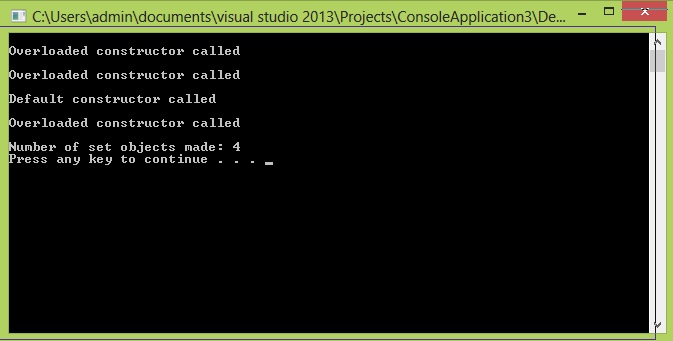# Static data members

In this part you will learn: 1. What is static data member? 2. Where static data members are used? 3. Syntax of Static data members 4. Writing program with Static data members 5. Showing output Static data member Static data members are those data members which retain their values, i.e. Static data members store the latest value in them so that the value will be used for next time when the function that makes use of static data member is called. In simple words, all the objects of a class share a common data member that is why we call it static. The most important and common use of using static data member is to count the number of times an object of a class is created, or more generally to count how many times a function is called. A static class member is initialized is usually initialized with 0 in case of integer type and NULL in case of character. Syntax class Class_Name { static int static_member_name; //declaration } int Class_Name :: static_member_name=0; //initialization to value 0 Note: As given in the above syntax, we have declared the static member inside the class. But its value is initialized outside the class. For this purpose we have first accessed the static member variable using scope resolution operator. Scope resolution operator (::) is a special type of operator used to access members of a class. Program with Static data member In this example I will illustrate how static data member work, for this purpose, let us take example of sets. Basic Step:
`#include<iostream>#include<conio.h>using namespace std;`
These two are the most common and basic lines of a c++ program. The first one iostream is used to grant us most of the basic functions of C++ like Input and Output functions. The second one conio.h means console input output, the black screen we see is the console. Using namespace std; provides us an ease of using all the library functions without specifying that this function belongs to standard library. Without including it, we have to write ‘std’ with all the built-in functions. Class
`class set{private:	static int count;public:	int *ele;	int size; 	set(){		cout << endl << "Default constructor called" << endl;		count = count + 1;		size = 0;		ele = NULL;		} 	set(int n){		cout << endl << "Overloaded constructor called" << endl;		count = count + 1;		size = n;		ele = new int [size];		} 	~set(){		cout << endl << "Destructor called" << endl;		if (size > 0)			delete[]ele;		ele = NULL;		}	int getcount(){		return count;		}}; int set::count = 0;`
The above given code is the definition of class “set” which has 2 member variables ele and size. There is also a static data member namely count. Then we have default and overloaded constructor and destructor. The point to note here is that I have added a statement in both the constructors to increase the value of count each time it is called. This is because I want to keep record of the number of times the default or overloaded constructor is called, in other words it will help us to know how many objects have been created. As we know that a constructor is called each time an object is created. Note: We could also have done this for copy constructor but for the sake of simplicity let us consider the case in which we won’t need a copy constructor. And we have a getter function at the end of class definition for count. In this function, we are returning the value of count which is a private member to the caller. After the definition of class, we have initialized the value of count to 0. We can assign it any value but for the current case we need it to be initialized at 0. The statement “int set::count = 0;” exactly means that There is an “int”(integer) type data which belongs to the class “set” namely “count”, assign it value 0. Note: Since we are outside the class definition so it is the responsibility of the programmer to specify the name of the class otherwise the compiler would generate an error. Main
`int main(){	set a(5);	set b(2);	set c;	set d(10); 	cout << endl << "Number of set objects made: " << a.getcount() << endl;	system("pause");} `
In the main program, we have simply made 4 different objects. Each time an object would be declared, the default or overloaded constructor would be called. It will increase the value of count. Since all the objects share a common variable (static variable), changes would be made for all the objects of set type. At the end I have printed the value of count using getcount() function. Note: I have called getcount() function using object “a”, you can call this function using any other object of this class. The result will be the same. Output